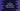# C program to separate even and odd numbers from an array in 3 ways## C program to separate even and odd numbers from an array and put them in different arrays:

In this example, we will learn how to separate odd and even numbers from an array and put it in different arrays with a `C` program. A number is called an even number if it is perfectly divisible by 2. Else, it is called an odd number. For example, 2, 4, 6, 8, etc. are Even numbers. I will show you different ways to write the `C` program in this post.

### Method 1: By using a `for` loop:

With this method, we will use one `for` loop to filter out the array elements. The program will use the following steps:

1. Suppose, the number array is already provided to the program.
2. We need to create two more arrays to store the odd and even numbers.
3. The program will run one loop and check for each number if it is divisible by 2 or not.
4. If yes, it will append that value to the array of even numbers. Else, it will append it to the array of odd numbers.
5. At the end of the program, it will print out the odd and even number arrays.

#### C program to separate even and odd numbers of an array with a `for` loop:

The following program shows how to separate the even and odd numbers of an array with a `for` loop:

``````#include <stdio.h>

int main()
{
// 1
int i, j, k;

// 2
int num = {1, 2, 3, 4, 5, 6, 7, 8, 9, 10};

// 3
int odd;
int even;

// 4
j = 0;
k = 0;

for (i = 0; i < 10; i++)
{
// 5
if (num[i] % 2 == 0)
{
even[j] = num[i];
j++;
}
else
{
odd[k] = num[i];
k++;
}
}

// 6
printf("Even numbers: ");
for (i = 0; i < j; i++)
{
printf("%d ", even[i]);
}

printf("\nOdd numbers: ");
for (i = 0; i < k; i++)
{
printf("%d ", even[i]);
}

printf("\n");
return 0;
}``````

#### Explanation:

The commented numbers in the above program denote the steps below :

1. Declare three integer variables `i`, `j`, and `k`.
2. The array `num` is the given array of integers. In this example, the size of this array is 10.
3. Initialize two integer arrays `odd` and `even` to hold the odd and even numbers of the array `num`.
4. Initialize `j` and `k` as 0. The integer variable `j` is used as the current index of the array `odd` and the variable `k` is used as the current index of the array `even`. It uses one `for` loop to iterate over the values of the `num` array.
5. For each element of `num`, it checks if it is `even` or `odd`. If it is `even`, it adds that value to the `even` array. Else, it adds it to the `odd` array. It also increments the value of `j` or `k` to point to the next position of the array.
6. At the end of the program, it prints the elements of the `even` and `odd` arrays.

#### Output :

``````Even numbers: 2 4 6 8 10
Odd numbers: 1 3 5 7 9``````

### Method 2: C program to separate even and odd numbers with user input values:

We can take the size of the array as input from the user before initializing the array. The following `C` program shows how to take the size of the array as input. This program will work for variable size arrays:

``````#include <stdio.h>

void printArray(int arr[], int length){
for(int i = 0; i < length; i++){
printf("%d ", arr[i]);
}
}

int main()
{
int i, j, k, size;

printf("Enter the size of the array: ");
scanf("%d", &size);

int num[size];
int odd[size];
int even[size];

for(i = 0; i < size; i++){
printf("Enter the number for position %d: ", i);
scanf("%d", &num[i]);
}

j = 0;
k = 0;

for (i = 0; i < size; i++)
{
if (num[i] % 2 == 0)
{
even[j] = num[i];
j++;
}
else
{
odd[k] = num[i];
k++;
}
}

printf("Even numbers: ");
printArray(even, j);

printf("\nOdd numbers: ");
printArray(odd, k);

printf("\n");
return 0;
}``````

• We created a new function `printArray`. This function takes one array and its length as the parameters and prints the content of the array. The main program calls this function to print the final array content.
• The size of the array is assigned to the `size` variable. It initializes the arrays with `size` length.

If you run this program, it will print similar output.

### Method 3: By using a `while` loop:

The following program shows how to use `while` loops to write the above program. The variable used in the loop is initialized before the loop starts and its value is incremented at the end of each iteration of the loop:

``````#include <stdio.h>

void printArray(int arr[], int length)
{
int i = 0;
while (i < length)
{
printf("%d ", arr[i]);
i++;
}
}

int main()
{
int i, j, k, size;

printf("Enter the size of the array: ");
scanf("%d", &size);

int num[size];
int odd[size];
int even[size];

i = 0;
while (i < size)
{
printf("Enter the number for position %d: ", i);
scanf("%d", &num[i]);
i++;
}

i = 0, j = 0, k = 0;

while (i < size)
{
if (num[i] % 2 == 0)
{
even[j] = num[i];
j++;
}
else
{
odd[k] = num[i];
k++;
}
i++;
}

printf("Even numbers: ");
printArray(even, j);

printf("\nOdd numbers: ");
printArray(odd, k);

printf("\n");
return 0;
}``````

The only difference in this program is that the `for` loops are replaced with `while` loops. The other parts of the program are not changed. It will print similar output.